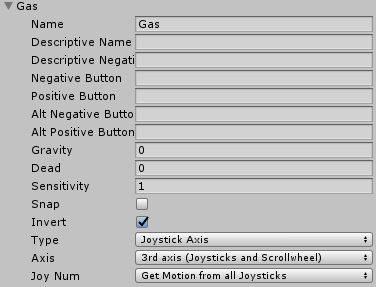# Logitech G27 pedal input

I’m trying to use Logitech G27 Gas pedal. When pedal isn’t pressed value is -100 and when it is pressed value is 100. Is there way to get value work between 0 and 100?

0 = pedal isn’t pressed.
100 = pedal is pressed.
_
My script looks like this:

``````using UnityEngine;
using System.Collections;

public class PedalTest : MonoBehaviour
{
public float Gas;
}

void Update()
{
Gas = Input.GetAxis("Gas") * 100;
}
}
``````

Input:_
``````gas = (Input.GetAxis("Gas") + 100) * 0.5f;